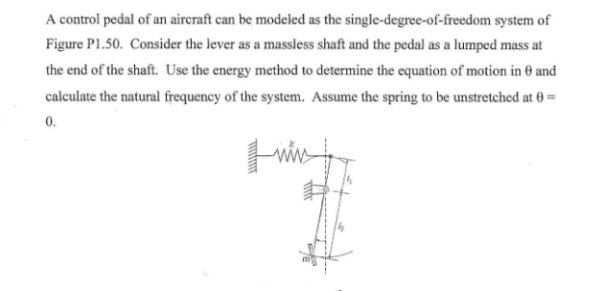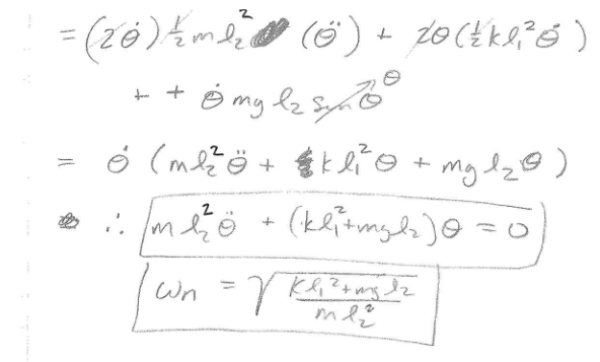A control pedal of an aircraft can be modeled as the single degree of freedom system of the figure. Consider the lever as a massless shaft and the pedal as a lumped mass at the end of the shaft. Use the energy method to determine the equation of motion in theta and calculate the natural frequency of the system. Assume the spring to be unstretched at theta=0.A control pedal of an aircraft can be modeled as the single degree of freedom system of the figure. Consider the lever as a massless shaft and the pedal as a lumped mass at the end of the shaft. Use the energy method to determine the equation of motion in theta and calculate the natural frequency of the system. Assume the spring to be unstretched at theta=0.

Vibrations Page 1 vibrations vibrations vibrations vibrations vibrations vibrations vibrations Vibrations Page 2